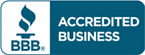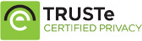# Test bank for College Algebra Graphs and Models 5th edition by Marvin L. Bittinger

\$30.00

College Algebra Graphs and Models 5th edition by Marvin L. Bittinger Test bank Questions

Full Chapters are included

Do you need Solution manual for this book?Categories: ,

## Test bank for College Algebra Graphs and Models 5th edition by Marvin L. Bittinger

Table of contents Test bank for College Algebra Graphs and Models 5th edition by Marvin L. Bittinger

R. Basic Concepts of Algebra
R.1 The Real-Number System
R.2 Integer Exponents, Scientific Notation, and Order of Operations
R.3 Addition, Subtraction, and Multiplication of Polynomials
R.4 Factoring
R.5 The Basics of Equation Solving
R.6 Rational Expressions
R.7 Radical Notation and Rational Exponents
1. Graphs, Functions, and Models
1.1 Introduction to Graphing
1.2 Functions and Graphs
1.3 Linear Functions, Slope, and Applications
1.4 Equations of Lines and Modeling
1.5 Linear Equations, Functions, Zeros, and Applications
1.6 Solving Linear Inequalities
2. More on Functions
2.1 Increasing, Decreasing, and Piecewise Functions; Applications
2.2 The Algebra of Functions
2.3 The Composition of Functions
2.4 Symmetry
2.5 Transformations
2.6 Variation and Applications
3. Quadratic Functions and Equations; Inequalities
3.1 The Complex Numbers
3.2 Quadratic Equations, Functions, Zeros, and Models
3.3 Analyzing Graphs of Quadratic Functions
3.4 Solving Rational Equations and Radical Equations
3.5 Solving Equations and Inequalities with Absolute Value
4. Polynomial and Rational Functions
4.1 Polynomial Functions and Modeling
4.2 Graphing Polynomial Functions
4.3 Polynomial Division; The Remainder Theorem and the Factor Theorem
4.4 Theorems about Zeros of Polynomial Functions
4.5 Rational Functions
4.6 Polynomial Inequalities and Rational Inequalities
5. Exponential and Logarithmic Functions
5.1 Inverse Functions
5.2 Exponential Functions and Graphs
5.3 Logarithmic Functions and Graphs
5.4 Properties of Logarithmic Functions
5.5 Solving Exponential and Logarithmic Equations
5.6 Applications and Models: Growth and Decay; Compound Interest
6. Systems of Equations and Matrices
6.1 Systems of Equations in Two Variables
6.2 Systems of Equations in Three Variables
6.3 Matrices and Systems of Equations
6.4 Matrix Operations
6.5 Inverses of Matrices
6.6 Determinants and Cramer’s Rule
6.7 Systems of Inequalities and Linear Programming
6.8 Partial Fractions
7. Conic Sections
7.1 The Parabola
7.2 The Circle and the Ellipse
7.3 The Hyperbola
7.4 Nonlinear Systems of Equations and Inequalities
8. Sequences, Series, and Combinatorics
8.1 Sequences and Series
8.2 Arithmetic Sequences and Series
8.3 Geometric Sequences and Series
8.4 Mathematical Induction
8.5 Combinatorics: Permutations
8.6 Combinatorics: Combinations
8.7 The Binomial Theorem
8.8 Probability
Photo Credits
Answers (Additional Instructor’s Answers) [in AIE only] Index of Applications
Index

## Reviews

There are no reviews yet.